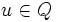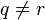# Corollary of centralizer product theorem for rank at least three

## Statement

Let$q,r,s$ be primes such that$q \ne r, q \ne s$ (we can have$r = s$).

Suppose$Q$ is a finite abelian$q$-group of rank at least three,$R$ is a finite$r$-group and$S$ is a finite$s$-group. Suppose further that$Q$ acts as automorphisms on both$R$ and$S$. Then, there exists a non-identity element$u \in Q$ such that$C_R(u)$ and$C_S(u)$ are both nontrivial.

## Facts used

The table below lists key facts used directly and explicitly in the proof. Fact numbers as used in the table may be referenced in the proof. This table need not list facts used indirectly, i.e., facts that are used to prove these facts, and it need not list facts used implicitly through assumptions embedded in the choice of terminology and language.
Fact no. Statement Steps in the proof where it is used Qualitative description of how it is used What does it rely on? Difficulty level Other applications
1 Centralizer product theorem (1), (4), (6) At each step, we have at hand a non-cyclic abelian group of prime power order acting on a group of a different prime power order, and we use the fact to show that the centralizer of at least one of the acting elements in the acted upon group is nontrivial. centralizer product theorem for elementary abelian group click here

## Proof

This proof uses a tabular format for presentation. Provide feedback on tabular proof formats in a survey (opens in new window/tab) | Learn more about tabular proof formats|View all pages on facts with proofs in tabular format

Given: A prime$q$, possibly equal primes$r,s$ both distinct from$q$. A finite abelian$q$-group$Q$ of rank at least three (in particular,$Q$ is non-cyclic). A finite$r$-group$R$ and a finite$s$-group$S$.

To prove: There exists a non-identity element$u$ of$Q$ such that both$C_R(u)$ and$C_S(u)$ are nontrivial.

Proof: Without loss of generality, we assume that$Q$ is elementary abelian of order$q^3$. This is because we can always replace$Q$ by an elementary abelian subgroup of order$q^3$ in$Q$, which exists because$Q$ has rank at least three.

Step no. Assertion/construction Facts used Given data used Previous steps used Explanation Commentary
1 There exists a non-identity element$x \in Q$ such that$C_R(x)$ is nontrivial. Fact (1)$Q$ is a non-cyclic abelian$q$-group ,$R$ is a$r$-group,$q \ne r$. Apply Fact (1) to the action of$Q$ on$R$. We're starting with a rank three group$Q$, which is more than what we need at this stage, since we only need rank at least two to guarantee an element with nontrivial centralizer. We have used up one piece of slack.
2 Let$R_0 = C_R(x)$. Then,$R_0$ is nontrivial and$Q$-invariant$Q$ is abelian. Step (1) [SHOW MORE] Process of quotienting out begins
3 Consider$Q_1 = Q/\langle x \rangle$. Then,$Q_1$ has rank two (being elementary abelian of order$q^2$), and the action of$Q$ on$R_0$ descends to an action of$Q_1$ on$R_0$.$Q$ is abelian Step (2) [SHOW MORE] Process of quotienting out continues
4 There exists$\overline{y} \in Q_1$ such that$C_{R_0}(\overline{y})$ is nontrivial. Fact (1) Step (3) [SHOW MORE] Having used up 1 of the rank 3 in Step (1), we are left with a rank 2 quotient acting on a subgroup of$R$. We can still use Fact (1) on this quotient of$Q$, to get a nontrivial centralizer within this.
5 Let$y$ be an element of$Q$ that maps to$\overline{y}$. Then,$C_{R_0}(y) = C_{R_0}(\overline{y})$ is nontrivial. Let$R_1 = C_{R_0}(y)$. Then, if$A$ is the elementary abelian subgroup$\langle x,y \rangle$ of$Q$,$C_R(u)$ contains the nontrivial group$R_1$ for all non-identity elements$u$ of$A$, and is hence nontrivial. Steps (2)-(4) [SHOW MORE] Now combining the previous steps to obtain a subgroup of$Q$ of rank two in which every element fixes a nontrivial subgroup of$R$.
6 There exists a non-identity element$u \in A$ such that$C_S(u)$ is nontrivial. Fact (1)$S$ is a$s$-group,$q \ne s$. Step (5) [SHOW MORE] We can use Fact (1) on this subgroup of$Q$ because it has rank at least 2, to find an element with nontrivial centralizer.
7 The$u \in A$ that works for Step (6) has the property that$u \in Q$ and both$C_R(u)$ and$C_S(u)$ are nontrivial. Steps (5), (6) [SHOW MORE] Combine steps.